# Variety of universal algebras

(diff) ← Older revision | Latest revision (diff) | Newer revision → (diff)

A class of universal algebras (cf. Universal algebra) defined by a system of identities (cf. Algebraic systems, variety of). A variety of universal algebras may be characterized as a non-empty class of algebras closed under taking quotient algebras, subalgebras and direct products. The last two conditions may be replaced by the requirement of closure under subdirect products. A variety of universal algebras is said to be trivial if it consists of one-element algebras. Every non-trivial variety of universal algebras contains a free algebra with basis of any cardinality. Ifandare bases of the same free algebra in a non-trivial variety andis infinite, thenandare equipotent. The requirement that one of the bases be infinite is essential, but it may be omitted if the variety contains a finite algebra with more than one element.

The variety of universal algebras generated by a classconsists of all quotient algebras of subdirect products of algebras in. If a variety of universal algebras is generated by finite algebras, then every finitely-generated algebra in the variety is finite. The congruences of any algebra in a variety of universal algebrasof signaturecommute if and only if there exists a ternary termof the signaturesuch that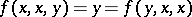for all algebras in. In similar fashion one can characterize varieties of universal algebras whose algebras have modular or distributive congruence lattices (cf. , , , ).

In a variety, an-ary operationis called trivial if for every algebra inthe identity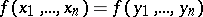holds. E.g. in the variety of rings with zero multiplication the operation of multiplication is trivial. Every trivial operationmay be replaced by the-ary operation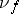defined by the equation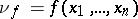. Suppose that the signatures,of two varieties of universal algebras,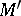, respectively, do not contain trivial operations. A mappingfrominto the set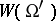of terms of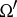is called admissible if the arities ofand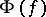coincide for all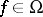. An admissible mappingcan be extended to a mapping from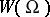to, still denoted by, in a natural fashion. The varietiesandare said to be rationally equivalent if there exist admissible mappings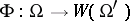andsuch thatfor all,for all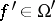, and if for every defining identity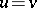(respectively,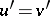) of(respectively,) the identity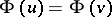(respectively,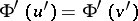) holds for all algebras in(in). The last requirement is equivalent to the fact that every algebrain(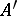in) corresponds to an algebra in(in), where each-ary operationin(in) is defined by the equation(respectively,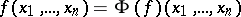). The variety of Boolean rings and that of Boolean algebras (cf. Boolean algebra) are rationally equivalent. The variety of unary algebras (cf. Unary algebra) of signature, with defining identities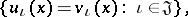is rationally equivalent to the variety of all left-polygons (cf. Polygon (over a monoid)), whereis the quotient monoid of the free monoid generated byby the congruence generated by the pairs. A variety of universal algebrasis rationally equivalent to the variety of all right modules over some associative ring if and only if the congruences on any algebra incommute, if finite free products (cf. Free product) incoincide with direct products (cf. Direct product) and if there exist-ary derived operations forming a distinguished subalgebra. The first two conditions may be replaced by the requirement: Every subalgebra of any algebra inis the class of a certain congruence and every congruence of any algebra inis uniquely determined by the class formed by the subalgebra, , .

The variety of lattices generated by the congruence lattices of all algebras of a certain variety of universal algebras is called a congruence variety. Not every variety of lattices is a congruence variety. There exist congruence varieties which are not modular and differ from the variety of all lattices , .

How to Cite This Entry:
Variety of universal algebras. Encyclopedia of Mathematics. URL: http://encyclopediaofmath.org/index.php?title=Variety_of_universal_algebras&oldid=16722
This article was adapted from an original article by L.A. Skornyakov (originator), which appeared in Encyclopedia of Mathematics - ISBN 1402006098. See original article